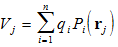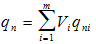Boundary Element Method equations.

(Taken from Chapter 1 of the Users Guide).

The technique that is used in the Boundary Element Method to determine the segment charges in non-space-charge simulations is also simple. The question that has to be answered is: what are the charges qi on the segments i (where i = 1 to n and n is the total number of segments) when the potentials Vi are applied to the segments (or more precisely, to the parent electrodes)? In the Boundary Element Method the problem is looked at from the reverse direction, and the question is asked: what are the potentials Vi that would result from a given set of charges qi? These potentials and charges are related through the linear equationwhere we take rj to be the mid-point of segment j, and where Pi(rj) is the potential at rj due to a unit charge on segment i. In other words, the potential Vj of segment j is due to the charges qi on all the segments i, including the self-potential due to the charge qj on the segment j itself. Clearly the quantities Pi(rj) can be calculated, essentially by using Coulomb`s Law. There are n of these equations, for j=1 to n. There are also n unknowns, qi, for i=1 to n. Nothing else is unknown, because all the segment parameters and hence all the functions Pi are (in principle) known. The n equations in n unknowns can therefore be solved to give the segment charges qi. The electrostatic problem is then completely solved. The system of electrodes has become a set of segments each of which carries a known charge distributed uniformly over its surface. Potentials and fields can now be calculated anywhere in space, again essentially by using Coulomb`s Law. Laplace`s equation, the equation for the electrostatic field, has therefore been solved.

After all the segments have been established, the charges on them are evaluated. More precisely, the unit charges are evaluated, which are the charges on the segments when each of the applied potentials is put equal to unity in turn while the remaining potentials are zero. These sets of charges are stored (in the binary processed data file) and used later to synthesise charges for any set of applied potentials. The synthesis is carried out as follows. Suppose that the applied potentials Vi is unity while all the other potentials Vj, where j = 1 to (i-1) or (i+1) to m, are zero, and suppose that the resulting charges on the segments are then qni, where n = 1 to N. The charges qni are the unit charges. Since the equations that connect charges and potentials are linear equations, the charge qn on segment n is given byfor a general set of potentials Vi.